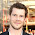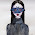## Monday, July 22, 2013

### Thread 1 cannot allocate new log & Checkpoint not complete

Users will see these messages when Oracle wants to reuse the redolog file, but checkpoint position is still in the log, Oracle must wait until the checkpoint completes.

Cause: In this situation either DBWR writes slowly or log switch happens before the log is completely full or log file is small.

Mon Apr 15 07:20:42 2013
Current log# 1 seq# 5021 mem# 0: /oracle/ORCL/redoA/redo01.log
Mon Apr 15 07:21:15 2013
Thread 1 cannot allocate new log, sequence 5022
Checkpoint not complete

If you have many updates in the system, you might need more redo groups. If you have fewer redo groups then adding more redo groups will help.

Use below syntax to add more redo groups

ALTER DATABASE ADD LOGFILE GROUP <Group No> ('<Redo log member 1 path’ ,'<redo log member 2 path>') size 500M;

If you have smaller redo log and if you see many log switches then increasing the redo size might help.

Step1: Switch logfile to make group 1 ‘INACTIVE’

SQL> Alter system switch logfile;
SQL> select group#, status from v\$log;

GROUP# STATUS
---------- ----------------
1 INACTIVE
2 ACTIVE
3 CURRENT

Step2:- Drop the log group1 which is ‘INACTIVE’ and recreate with bigger size.

SQL> alter database drop logfile group 1;

SQL> alter database add logfile group 1 ('/db01/ORCL/redoA/log1_m1.dbf',' /db01/ORCL/redoB/log1_m2.dbf') size 100M reuse;

Repeat step 1 and 2 until you drop and recreate all redo logs with bigger size.

It is a recommended to have 4-5 log switches per hour. You can use below Script to find the log switches on hourly basis.

set lines 120;
set pages 999;
SELECT to_char(first_time,'YYYY-MON-DD') day,
to_char(sum(decode(to_char(first_time,'HH24'),'00',1,0)),'99') "00",
to_char(sum(decode(to_char(first_time,'HH24'),'01',1,0)),'99') "01",
to_char(sum(decode(to_char(first_time,'HH24'),'02',1,0)),'99') "02",
to_char(sum(decode(to_char(first_time,'HH24'),'03',1,0)),'99') "03",
to_char(sum(decode(to_char(first_time,'HH24'),'04',1,0)),'99') "04",
to_char(sum(decode(to_char(first_time,'HH24'),'05',1,0)),'99') "05",
to_char(sum(decode(to_char(first_time,'HH24'),'06',1,0)),'99') "06",
to_char(sum(decode(to_char(first_time,'HH24'),'07',1,0)),'99') "07",
to_char(sum(decode(to_char(first_time,'HH24'),'08',1,0)),'99') "0",
to_char(sum(decode(to_char(first_time,'HH24'),'09',1,0)),'99') "09",
to_char(sum(decode(to_char(first_time,'HH24'),'10',1,0)),'99') "10",
to_char(sum(decode(to_char(first_time,'HH24'),'11',1,0)),'99') "11",
to_char(sum(decode(to_char(first_time,'HH24'),'12',1,0)),'99') "12",
to_char(sum(decode(to_char(first_time,'HH24'),'13',1,0)),'99') "13",
to_char(sum(decode(to_char(first_time,'HH24'),'14',1,0)),'99') "14",
to_char(sum(decode(to_char(first_time,'HH24'),'15',1,0)),'99') "15",
to_char(sum(decode(to_char(first_time,'HH24'),'16',1,0)),'99') "16",
to_char(sum(decode(to_char(first_time,'HH24'),'17',1,0)),'99') "17",
to_char(sum(decode(to_char(first_time,'HH24'),'18',1,0)),'99') "18",
to_char(sum(decode(to_char(first_time,'HH24'),'19',1,0)),'99') "19",
to_char(sum(decode(to_char(first_time,'HH24'),'20',1,0)),'99') "20",
to_char(sum(decode(to_char(first_time,'HH24'),'21',1,0)),'99') "21",
to_char(sum(decode(to_char(first_time,'HH24'),'22',1,0)),'99') "22",
to_char(sum(decode(to_char(first_time,'HH24'),'23',1,0)),'99') "23"
from
v\$log_history
GROUP by to_char(first_time,'YYYY-MON-DD');

Regards,
Satishbabu Gunukula, Oracle ACE
http://www.oracleracexpert.com

1.Hello Satish,

Will this scenario is a reason for primary and standby not in sync??

2.3.4.5.